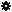﻿ Object Model: PolygonFaceCollection.GrowNeighborPolygons

PolygonFaceCollection.GrowNeighborPolygons

## PolygonFaceCollection.GrowNeighborPolygonsv3.0

### Description

Returns a collection of PolygonFace objects for all polygons that are adjacent to each Vertex of each PolygonFace in this collection, within a given distance.

### C# Syntax

 ```PolygonFaceCollection PolygonFaceCollection.GrowNeighborPolygons( Int32 in_lDistance ); ```

### Scripting Syntax

 ```oReturn = PolygonFaceCollection.GrowNeighborPolygons( [Distance] ); ```

### Parameters

Parameter Type Description
Distance Integer Distance is an integer value representing the degree of neighborhood (eg: degree=2 for a polygon means its adjacent polygons plus the adjacent polygons of the adjacent polygons)

Default Value: 1

### Examples

#### VBScript Example

 ```set oCube = ActiveSceneRoot.AddGeometry( "Cone", "MeshSurface" ) set oGeometry = oCube.ActivePrimitive.Geometry set oSubComponent = oGeometry.CreateSubComponent(siPolygonCluster,Array(1,2)) set oPolygons = oSubComponent.ComponentCollection set oNeighborPolygons = oPolygons.GrowNeighborPolygons str = "Polygons around the polygons 1 and 2 are :" for each n in oNeighborPolygons str = str & " " & n.index next logmessage str ```